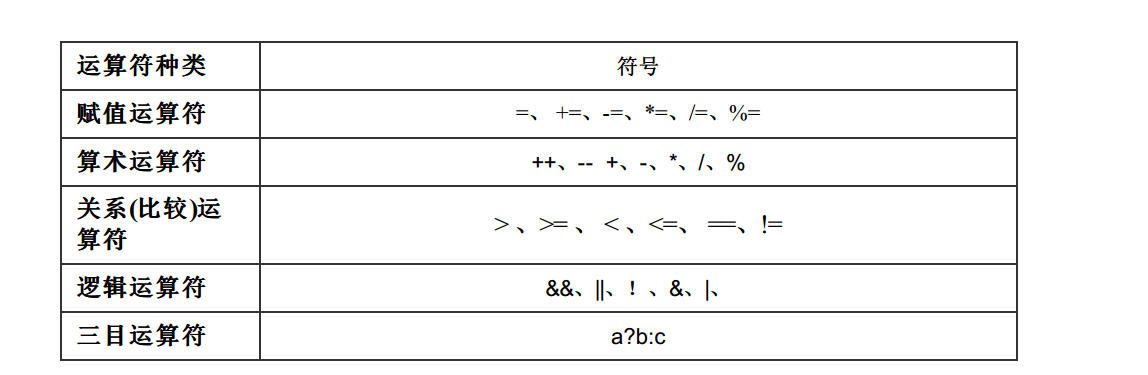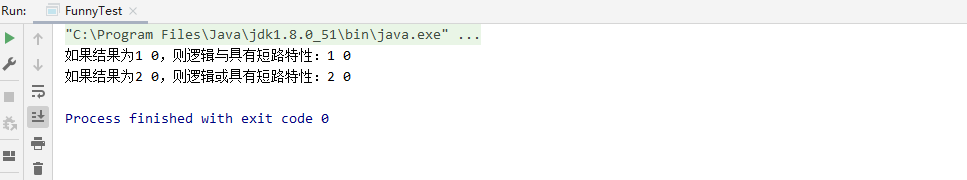# 这道逻辑运算符的面试题难吗？## 面试题

``````public class Test{
public static void main(String[] args){
int x = 0;
int y = 0;
int k = 0;
for (int z = 0; z < 5; z++) {
if ((++x > 2) && (++y > 2) && (k++ > 2))
{
x++;
++y;
k++;
}
}
System.out.println(x + "" + y + "" + k);
}
}``````

``A.432    B.531    C.421    D.523``

## 考查知识点``````//需要验证的内容如下：

if(a && b)    //如果 a 为 false, Java还会校验 b 吗？

if(a || b)    //如果 a 为 true, Java还会校验 b 吗？``````

## 实践出真知

``````public class FunnyTest{
public static void main(String[] args){
int a = 0;
int b = 0;

if((a++ > 0) && (b++ > 0)) ;

System.out.println("如果结果为1 0，则逻辑与具有短路特性：" + a +" " + b);

//此时a = 1, b = 0

if((a++ > 0) || (b++ > 0)) ;

System.out.println("如果结果为2 0，则逻辑或具有短路特性：" + a +" " + b);
}
}``````a, b 的初始值均为 0，

## 回归题目

（注意：5次循环都没有满足if条件）

## 引申思考

``````if (str != null && !str.isEmpty()) {
doSomethingWith(str);
}``````

``````if (str == null || str.isEmpty()) {
unusableString();
} else {
doSomethingWith(str);
}``````